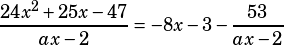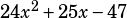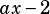Test 3 Section 3 Number 13 please!

OK, so this is one of those questions that looks way worse than it actually is. Here’s what you’re given:That’s just another way of saying that then the polynomialis divided by the polynomial, the quotient isand the remainder is.

Now, here’s the important part. If you think about the first step in polynomial division, it’s this: you divide the leading coefficient of the dividend by the leading coefficient of the divisor. In this case, that means you divide 24 by a. And that tells you the leading coefficient of the quotient, which is –8. What’s the only number you can divide 24 by to get –8? That’s right, a MUST equal –3.

That’s it—you’re done! I’m going to put the full division below just because I like doing math, but in a timed test situation you should stop right there and bubble in B.